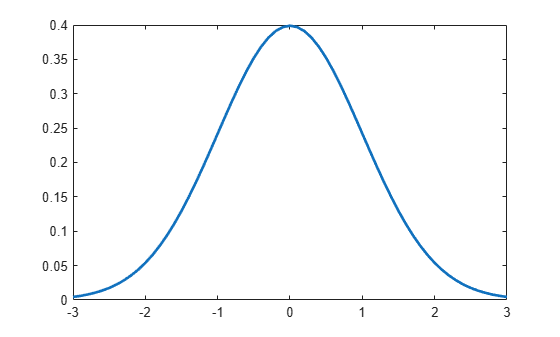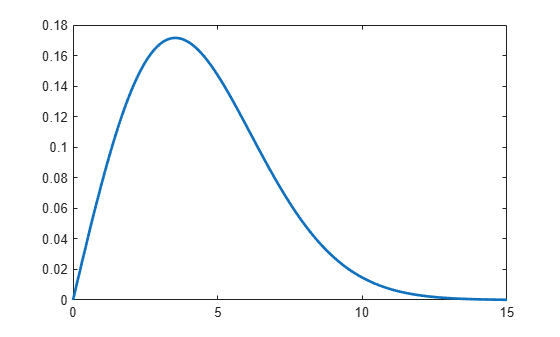# pdf

Probability density function

## Syntax

``y = pdf(name,x,A)``
``y = pdf(name,x,A,B)``
``y = pdf(name,x,A,B,C)``
``y = pdf(name,x,A,B,C,D)``
``y = pdf(pd,x)``

## Description

````y = pdf(name,x,A)` returns the probability density function (pdf) for the one-parameter distribution family specified by `name` and the distribution parameter `A`, evaluated at the values in `x`.```

example

````y = pdf(name,x,A,B)` returns the pdf for the two-parameter distribution family specified by `name` and the distribution parameters `A` and `B`, evaluated at the values in `x`.```
````y = pdf(name,x,A,B,C)` returns the pdf for the three-parameter distribution family specified by `name` and the distribution parameters `A`, `B`, and `C`, evaluated at the values in `x`.```
````y = pdf(name,x,A,B,C,D)` returns the pdf for the four-parameter distribution family specified by `name` and the distribution parameters `A`, `B`, `C`, and `D`, evaluated at the values in `x`.```

example

````y = pdf(pd,x)` returns the pdf of the probability distribution object `pd`, evaluated at the values in `x`.```

## Examples

collapse all

Compute the pdf values for a normal distribution by specifying the distribution name `'Normal'` and the distribution parameters.

Define the input vector `x` to contain the values at which to calculate the pdf.

`x = [-2 -1 0 1 2];`

Compute the pdf values for the normal distribution with the mean $\mu$ equal to 1 and the standard deviation $\sigma$ equal to 5.

```mu = 1; sigma = 5; y = pdf('Normal',x,mu,sigma)```
```y = 1×5 0.0666 0.0737 0.0782 0.0798 0.0782 ```

Each value in `y` corresponds to a value in the input vector `x`. For example, at the value `x` equal to 1, the corresponding pdf value `y` is equal to 0.0798.

Create a normal distribution object and compute the pdf values of the normal distribution using the object.

Create a normal distribution object with the mean $\mu$ equal to 1 and the standard deviation $\sigma$ equal to 5.

```mu = 1; sigma = 5; pd = makedist('Normal','mu',mu,'sigma',sigma);```

Define the input vector `x` to contain the values at which to calculate the pdf.

`x = [-2 -1 0 1 2];`

Compute the pdf values for the normal distribution at the values in x.

`y = pdf(pd,x)`
```y = 1×5 0.0666 0.0737 0.0782 0.0798 0.0782 ```

Each value in `y` corresponds to a value in the input vector `x`. For example, at the value `x` equal to 1, the corresponding pdf value `y` is equal to 0.0798.

Create a Poisson distribution object with the rate parameter, $\lambda$, equal to 2.

```lambda = 2; pd = makedist('Poisson','lambda',lambda);```

Define the input vector x to contain the values at which to calculate the pdf.

`x = [0 1 2 3 4];`

Compute the pdf values for the Poisson distribution at the values in x.

`y = pdf(pd,x)`
```y = 1×5 0.1353 0.2707 0.2707 0.1804 0.0902 ```

Each value in y corresponds to a value in the input vector x. For example, at the value x equal to 3, the corresponding pdf value in y is equal to 0.1804.

Alternatively, you can compute the same pdf values without creating a probability distribution object. Use the `pdf` function, and specify a Poisson distribution using the same value for the rate parameter, $\lambda$.

`y2 = pdf('Poisson',x,lambda)`
```y2 = 1×5 0.1353 0.2707 0.2707 0.1804 0.0902 ```

The pdf values are the same as those computed using the probability distribution object.

Create a standard normal distribution object.

`pd = makedist('Normal')`
```pd = NormalDistribution Normal distribution mu = 0 sigma = 1 ```

Specify the `x` values and compute the pdf.

```x = -3:.1:3; pdf_normal = pdf(pd,x);```

Plot the pdf.

`plot(x,pdf_normal,'LineWidth',2)`Create a Weibull probability distribution object.

`pd = makedist('Weibull','A',5,'B',2)`
```pd = WeibullDistribution Weibull distribution A = 5 B = 2 ```

Specify the `x` values and compute the pdf.

```x = 0:.1:15; y = pdf(pd,x);```

Plot the pdf.

`plot(x,y,'LineWidth',2)`## Input Arguments

collapse all

Probability distribution name, specified as one of the probability distribution names in this table.

`name`DistributionInput Parameter `A`Input Parameter `B`Input Parameter `C`Input Parameter `D`
`'Beta'`Beta Distributiona first shape parameterb second shape parameterN/AN/A
`'Binomial'`Binomial Distributionn number of trialsp probability of success for each trialN/AN/A
`'BirnbaumSaunders'`Birnbaum-Saunders Distributionβ scale parameterγ shape parameterN/AN/A
`'Burr'`Burr Type XII Distributionα scale parameterc first shape parameterk second shape parameterN/A
`'Chisquare'` or `'chi2'`Chi-Square Distributionν degrees of freedomN/AN/AN/A
`'Exponential'`Exponential Distributionμ meanN/AN/AN/A
`'Extreme Value'` or `'ev'`Extreme Value Distributionμ location parameterσ scale parameterN/AN/A
`'F'`F Distributionν1 numerator degrees of freedomν2 denominator degrees of freedomN/AN/A
`'Gamma'`Gamma Distributiona shape parameterb scale parameterN/AN/A
```'Generalized Extreme Value'``` or `'gev'`Generalized Extreme Value Distributionk shape parameterσ scale parameterμ location parameterN/A
`'Generalized Pareto'` or `'gp'`Generalized Pareto Distributionk tail index (shape) parameterσ scale parameterμ threshold (location) parameterN/A
`'Geometric'`Geometric Distributionp probability parameterN/AN/AN/A
`'Half Normal'` or `'hn'`Half-Normal Distributionμ location parameterσ scale parameterN/AN/A
`'Hypergeometric'` or `'hyge'`Hypergeometric Distributionm size of the populationk number of items with the desired characteristic in the populationn number of samples drawnN/A
`'InverseGaussian'`Inverse Gaussian Distributionμ scale parameterλ shape parameterN/AN/A
`'Logistic'`Logistic Distributionμ meanσ scale parameterN/AN/A
`'LogLogistic'`Loglogistic Distributionμ mean of logarithmic valuesσ scale parameter of logarithmic valuesN/AN/A
`'LogNormal'`Lognormal Distributionμ mean of logarithmic valuesσ standard deviation of logarithmic valuesN/AN/A
`'Loguniform'`Loguniform Distributiona lower endpoint (minimum)b upper endpoint (maximum)N/AN/A
`'Nakagami'`Nakagami Distributionμ shape parameterω scale parameterN/AN/A
`'Negative Binomial'` or `'nbin'`Negative Binomial Distributionr number of successesp probability of success in a single trialN/AN/A
`'Noncentral F'` or `'ncf'`Noncentral F Distributionν1 numerator degrees of freedomν2 denominator degrees of freedomδ noncentrality parameterN/A
`'Noncentral t'` or `'nct'`Noncentral t Distributionν degrees of freedomδ noncentrality parameterN/AN/A
`'Noncentral Chi-square'` or `'ncx2'`Noncentral Chi-Square Distributionν degrees of freedomδ noncentrality parameterN/AN/A
`'Normal'`Normal Distributionμ mean σ standard deviationN/AN/A
`'Poisson'`Poisson Distributionλ meanN/AN/AN/A
`'Rayleigh'`Rayleigh Distributionb scale parameterN/AN/AN/A
`'Rician'`Rician Distributions noncentrality parameterσ scale parameterN/AN/A
`'Stable'`Stable Distributionα first shape parameterβ second shape parameterγ scale parameterδ location parameter
`'T'`Student's t Distributionν degrees of freedomN/AN/AN/A
`'tLocationScale'`t Location-Scale Distributionμ location parameterσ scale parameterν shape parameterN/A
`'Uniform'`Uniform Distribution (Continuous)a lower endpoint (minimum)b upper endpoint (maximum)N/AN/A
`'Discrete Uniform'` or `'unid'`Uniform Distribution (Discrete)n maximum observable valueN/AN/AN/A
`'Weibull'` or `'wbl'`Weibull Distributiona scale parameterb shape parameterN/AN/A

Example: `'Normal'`

Values at which to evaluate the pdf, specified as a scalar value or an array of scalar values.

If one or more of the input arguments `x`, `A`, `B`, `C`, and `D` are arrays, then the array sizes must be the same. In this case, `pdf` expands each scalar input into a constant array of the same size as the array inputs. See `name` for the definitions of `A`, `B`, `C`, and `D` for each distribution.

Example: `[-1,0,3,4]`

Data Types: `single` | `double`

First probability distribution parameter, specified as a scalar value or an array of scalar values.

If one or more of the input arguments `x`, `A`, `B`, `C`, and `D` are arrays, then the array sizes must be the same. In this case, `pdf` expands each scalar input into a constant array of the same size as the array inputs. See `name` for the definitions of `A`, `B`, `C`, and `D` for each distribution.

Data Types: `single` | `double`

Second probability distribution parameter, specified as a scalar value or an array of scalar values.

If one or more of the input arguments `x`, `A`, `B`, `C`, and `D` are arrays, then the array sizes must be the same. In this case, `pdf` expands each scalar input into a constant array of the same size as the array inputs. See `name` for the definitions of `A`, `B`, `C`, and `D` for each distribution.

Data Types: `single` | `double`

Third probability distribution parameter, specified as a scalar value or an array of scalar values.

If one or more of the input arguments `x`, `A`, `B`, `C`, and `D` are arrays, then the array sizes must be the same. In this case, `pdf` expands each scalar input into a constant array of the same size as the array inputs. See `name` for the definitions of `A`, `B`, `C`, and `D` for each distribution.

Data Types: `single` | `double`

Fourth probability distribution parameter, specified as a scalar value or an array of scalar values.

If one or more of the input arguments `x`, `A`, `B`, `C`, and `D` are arrays, then the array sizes must be the same. In this case, `pdf` expands each scalar input into a constant array of the same size as the array inputs. See `name` for the definitions of `A`, `B`, `C`, and `D` for each distribution.

Data Types: `single` | `double`

Probability distribution, specified as one of the probability distribution objects in this table.

Distribution ObjectFunction or App to Create Probability Distribution Object
`BetaDistribution``makedist`, `fitdist`, Distribution Fitter
`BinomialDistribution``makedist`, `fitdist`, Distribution Fitter
`BirnbaumSaundersDistribution``makedist`, `fitdist`, Distribution Fitter
`BurrDistribution``makedist`, `fitdist`, Distribution Fitter
`ExponentialDistribution``makedist`, `fitdist`, Distribution Fitter
`ExtremeValueDistribution``makedist`, `fitdist`, Distribution Fitter
`GammaDistribution``makedist`, `fitdist`, Distribution Fitter
`GeneralizedExtremeValueDistribution``makedist`, `fitdist`, Distribution Fitter
`GeneralizedParetoDistribution``makedist`, `fitdist`, Distribution Fitter
`HalfNormalDistribution``makedist`, `fitdist`, Distribution Fitter
`InverseGaussianDistribution``makedist`, `fitdist`, Distribution Fitter
`KernelDistribution``fitdist`, Distribution Fitter
`LogisticDistribution``makedist`, `fitdist`, Distribution Fitter
`LoglogisticDistribution``makedist`, `fitdist`, Distribution Fitter
`LognormalDistribution``makedist`, `fitdist`, Distribution Fitter
`LoguniformDistribution``makedist`
`MultinomialDistribution``makedist`
`NakagamiDistribution``makedist`, `fitdist`, Distribution Fitter
`NegativeBinomialDistribution``makedist`, `fitdist`, Distribution Fitter
`NormalDistribution``makedist`, `fitdist`, Distribution Fitter
Piecewise distribution with generalized Pareto distributions in the tails`paretotails`
`PiecewiseLinearDistribution``makedist`
`PoissonDistribution``makedist`, `fitdist`, Distribution Fitter
`RayleighDistribution``makedist`, `fitdist`, Distribution Fitter
`RicianDistribution``makedist`, `fitdist`, Distribution Fitter
`StableDistribution``makedist`, `fitdist`, Distribution Fitter
`tLocationScaleDistribution``makedist`, `fitdist`, Distribution Fitter
`TriangularDistribution``makedist`
`UniformDistribution``makedist`
`WeibullDistribution``makedist`, `fitdist`, Distribution Fitter

## Output Arguments

collapse all

pdf values, returned as a scalar value or an array of scalar values. `y` is the same size as `x` after any necessary scalar expansion. Each element in `y` is the pdf value of the distribution, specified by the corresponding elements in the distribution parameters (`A`, `B`, `C`, and `D`) or specified by the probability distribution object (`pd`), evaluated at the corresponding element in `x`.

## Alternative Functionality

• `pdf` is a generic function that accepts either a distribution by its name `name` or a probability distribution object `pd`. It is faster to use a distribution-specific function, such as `normpdf` for the normal distribution and `binopdf` for the binomial distribution. For a list of distribution-specific functions, see Supported Distributions.

• Use the Probability Distribution Function app to create an interactive plot of the cumulative distribution function (cdf) or probability density function (pdf) for a probability distribution.

## Version History

Introduced before R2006a